# Excel Formulas for Calculating the Volume of Storm Water Detention Ponds

## Storm Water Detention/Retention Pond Background

When development takes place on a watershed, the amount of storm water runoff and the peak runoff rate typically increase from the pre-development values. The watershed runoff coefficient typically increases and the watershed time of concentration usually decreases due to development. These two factors both contribute to more storm water runoff and a higher peak rate of storm water runoff.

Many state and local agencies require storm water detention facilities that limit the rate of storm water runoff to the pre-development value whenever development takes place. Detention ponds and retention ponds are both facilities that capture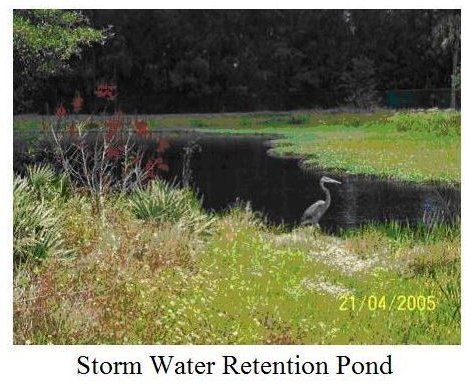storm water runoff and release it at the pre-development runoff rate or less. A detention pond doesn’t hold water between storm events, while a retention pond retains water in the pond between storm events.

For more background on storm water retention/detention ponds and the need for them, see the article, "The Stormwater Detention Pond and Stormwater Retention Pond – Why Are They Needed?" The rest of this article is devoted to the first step in design of storm water retention and storm water detention ponds, that is, determining the volume requirement. The parameters and equations will be presented along with downloadable spreadsheets having Excel formulas for making the calculations.

Image Credit: Brevard County Florida Watershed Management Program

## Parameters for calculating Volume Needed for Storm Water Detention Ponds

The watershed parameters needed for the storm water detention pond volume calculations considered in this article are as follows: (NOTE: The subscript, b, refers to before development watershed conditions and the subscript, a, refers to after development watershed conditions.)

• watershed area, A, acres
• storm water runoff depth – before, Qb, inches
• storm water runoff depth – after, Qa, inches
• peak storm water runoff rate – before, qpb, cfs
• peak storm water runoff rate – after, qpa, cfs
• runoff rate ratio, α, (α = qpb/qpa), dimensionless
• watershed time of concentration – before, tcb, hours
• watershed time of concentration – after, tca, hours
• time ratio, γ (γ = tcb/tca), dimensionless

Read on for the equations used in several methods of calculating volume needed for storm water detention ponds.

## Equations for Calculating Volume Needed for Storm Water Detention Ponds

Many methods have been proposed and are in use for estimating the volume requirement for storm water detention/retention ponds. Reference #1 for this article describes 7 methods. Three of them will be presented and discussed here as follows:

• the Generalized Model
• the Natural Storage Lost Model
• the Rational Hydrograph Model

The equation(s) used for each of them are as follows:

The Generalized Model equations are

• Vs = Qa {[γ + α + αγ(g + α – 4)]/(γ – α)} for α < 2 – γ
• Vs = Qa (γ – α)/(γ + α) for α > 2 – γ

where Vs is the storage volume needed for the detention/retention pond in inches over the watershed area and the other parameters are as defined above.

The Natural Storage Model equation is simply: Vs = Qa – Qb.

The Rational Hydrograph Model equation is: Vst = 0.08264 tca (qpa – qpb)

Go to page 2 for further discussion of each of these methods and Excel spreadsheets that can be downloaded to make the calculations in either U.S. or S.I. units.

## Excel Formulas for Detention Volume Calculation with the Generalized Model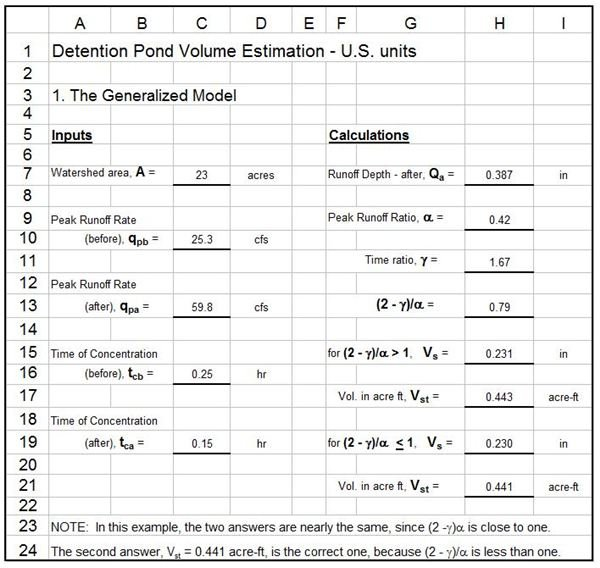The Excel spreadsheet image at the left shows the inputs needed and the calculations made to get an estimate of the detention pond volume requirement for a given watershed. The calculations use Excel formulas for the Generalized Model equations shown in the box at the right. The equations in the box give the detention pond volume, Vs, as a function of α, γ, and Qa.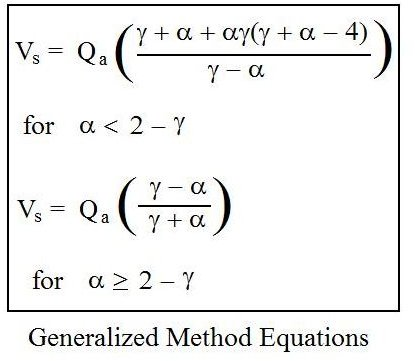In order to calculate values for the peak runoff ratio, α, and the time ratio, γ, values are needed for qpb, qpa, tcb, and tca.

For information about determining time of concentration from watershed characteristics and Excel spreadsheets that can be downloaded to make the calculations, see the article, "Excel Spreadsheet Templates for Calculating Watershed Time of Concentration." The peak runoff rates, qpb and qpa, would typically be calculated using the rational method as described in the article, "The Rational Method for Calculation of Peak Storm Water Runoff Rate," and the other articles in the series with it.

The runoff depth after development, Qa, can be calculated from the equation, Qa = (120/121)(qpa tca/A). Also, the detention pond volume, Vs, as calculated from the Generalized model is in inches over the watershed area. This volume can be converted to the more usable units, acre-ft, with the equation: Vst = Vs A/12, giving Vst in acre-ft, if Vs is in inches and A is in acres. The S.I. versions of these equations are presented in a later section on page 3 of this article.

## Loss of Natural Storage Detention Pond Volume Calculations with Excel Formulas

The Excel formulas in the spreadsheet image at the right calculate storm water detention pond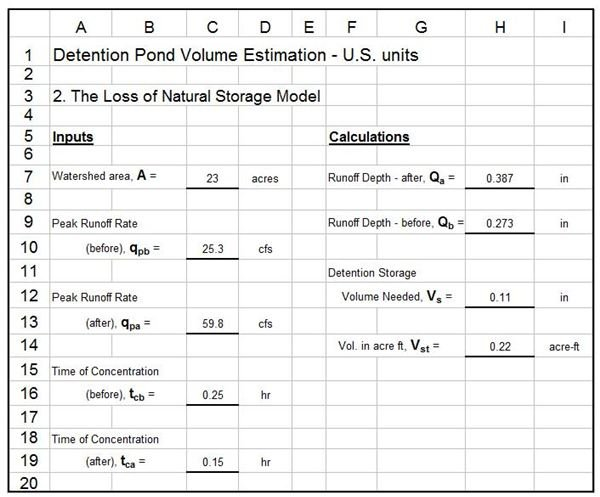volume with the loss of natural storage model. The input parameters needed for this model are the same as those needed for the Generalized model discussed in the last section, A, qpb, qpa, tcb, and tca. The last four of these parameters are needed to calculate Qb and Qa. Then the required detention pond storage in inches is Vs = Qb – Qa. The watershed area, A, is then needed to calculate the required detention pond volume in acre-ft, using the equation, Vst = Vs A/12.

Information about calculating peak storm water runoff rate and time of concentration can be found in the articles mentioned above in the previous section. The S.I. versions of these equations are given in a later section of this article on page 3.

## Detention Pond Volume Calculation by the Rational Hydrograph Model with Excel Formulas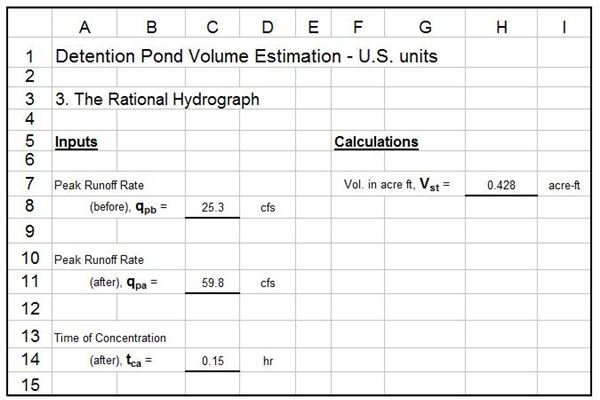The spreadsheet image at the left shows the calculation of storm water detention pond volume requirement with Excel formulas using the Rational Hydrograph model. This model is the simplest of the three discussed in this article. Only three input parameters are needed, pre-development peak runoff rate, qpb, after development peak runoff rate, qpa, and after development time of concentration, tca. Then the single equation, Vst = 0.08264 tca( qpa – qpb ), can be used to calculate the detention pond volume requirement directly in acre-ft. The S.I. version of this equation is given in the next section.

## Equations and Parameters For Detention Pond Volume Calculations in S.I. Units

The parameters for storm water detention pond volume calculations in S.I. units are as follows:

• watershed area, A, ha• storm water runoff depth – before, Qb, mm
• storm water runoff depth – after, Qa, mm
• peak storm water runoff rate – before, qpb, m3/s
• peak storm water runoff rate – after, qpa, m3/s
• runoff rate ratio, α, (α = qpb/qpa), dimensionless
• watershed time of concentration – before, tcb, hours
• watershed time of concentration – after, tca, hours
• time ratio, γ (γ = tcb/tca), dimensionless

The equations for the Generalized Model (shown in the box at the right above) remain the same for either U.S. or S.I. units, because α and γ are dimensionless. In U.S. units, both Qa and Vs are in inches, while in S.I. units, both Qa and Vs are in mm. The equation for converting detention pond volume in mm over the watershed area, Vs, to m3, is: Vst = (10)(Vs)(A) m3. In S.I. units the runoff depth, Qb or Qa, can be calculated from known values for the peak runoff rate, time of concentration, and watershed area, with the equation: Q – 360(qp tc/A). A in ha, qp in m3/s, and tc in hr, will give Q in mm.

The basic equation for the Loss of Natural Storage Model: Vs = Qa – Qb, remains the same for S.I. units. For S.I. calculations, all three parameters in the equation will be in mm instead of inches, as they were for U.S. units. The equations for Vst and Qa or Qb remain the same as shown above for the Generalized Model.

The constant in the Rational Hydrograph Model equation changes for S.I. units to become: Vst = 3600 tca (qpa – qpb). This equation will give Vst in m3, with units of hr for tca and m3/s for qpa and qpb.

The S.I. version spreadsheets that can be downloaded from previous sections of this article use these equations in the Excel formulas that make the calculations. The equations and units are also shown on the downloadable spreadsheets.

## References

References for Further Information:

1. McCuen, Richard H., Hydrologic Analysis and Design, 2nd Ed, Upper Saddle River, NJ, 1998.

2. Coefield, S. and Environmental Health News, "EPA to Staunch Flood of Storm Water Runoff Polluting U.S. Waterways," Scientific American, February 17, 2010.

3. Mays, L. W., Urban Stormwater Management Tools, New York, McGraw-Hill, 2004.

## This post is part of the series: Storm Water Retention and Detention Ponds

Storm water retention/detention ponds are needed on a developed watershed to capture storm water runoff and release it at a rate no greater than the pre-development peak storm water runoff rate. Time of concentration and runoff coefficient are important watershed parameters